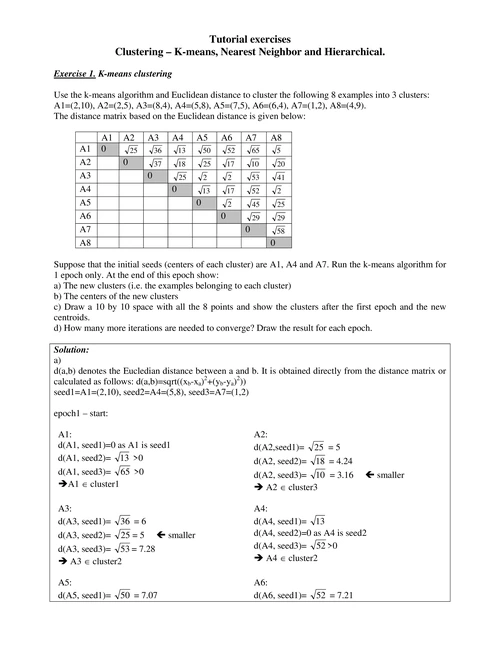### Tutorial exercises Clustering ? K-means, Nearest Neighbor and

Corrigé. Exercice 1 (03 points) : a/ Expliquez le principe d'une classification KMeans. (1.5 points). Exercice 2 (07 points) : Le tableau suivant contient des

#### Part of the document

Tutorial exercises
Clustering - K-means, Nearest Neighbor and Hierarchical.

Exercise 1.
K-means clustering

Use the k-means algorithm and Euclidean distance to cluster the following 8 examples into 3 clusters:
A1=(2,10), A2=(2,5), A3=(8,4), A4=(5,8), A5=(7,5), A6=(6,4), A7=(1,2), A8=(4,9).
The distance matrix based on the Euclidean distance is given below:

Suppose that the initial seeds (centers of each cluster) are A1, A4 and A7. Run the k-means algorithm for
1 epoch only. At the end of this epoch show:
a) The new clusters (i.e. the examples belonging to each cluster)
b) The centers of the new clusters
c) Draw a 10 by 10 space with all the 8 points and show the clusters after the first epoch and the new
centroids.
d) How many more iterations are needed to converge? Draw the result for each epoch.

Solution:
a)
d(a,b) denotes the Eucledian distance between a and b. It is obtained directly from the distance matrix or
calculated as follows: d(a,b)=sqrt((xb